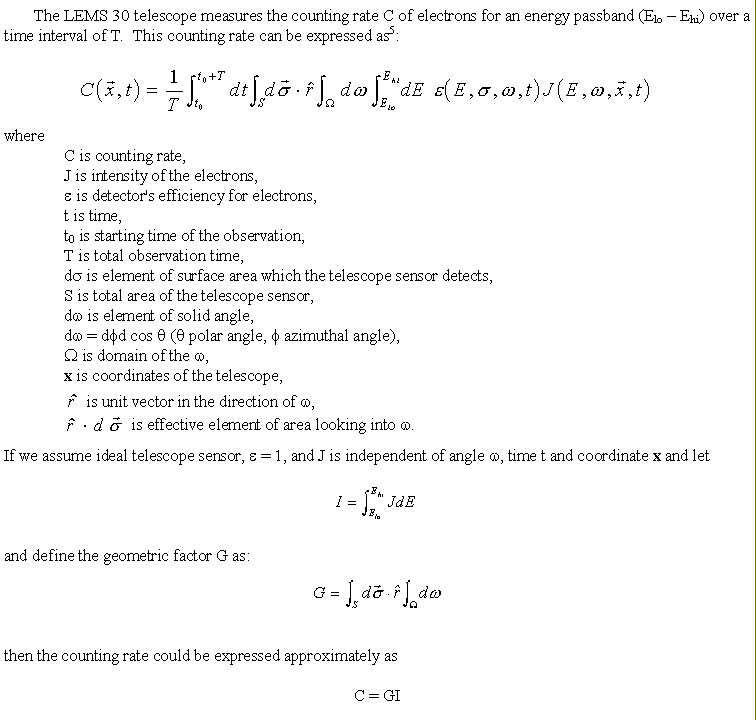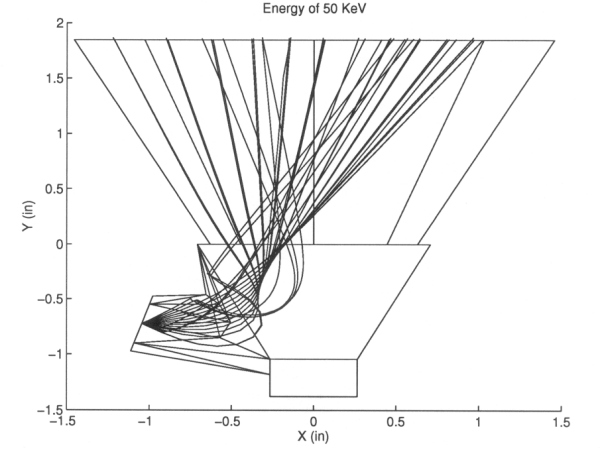ULYSSES

Ulysses HISCALE Data Analysis Handbook

## Appendix 10. Effect of Backscattered Electrons on the Geometric Factors of the LEMS30 Telescope (Hong MS Thesis)

### A10.2 Chapter 2 - Geometric FactorHence a known geometric factor is necessary to convert counting rate into particle flux which is the main objective of the telescope.

The geometric factor depends on the mechanical structure of the telescope, the magnetic field present in the telescope system and particle mass, charge and energy. For example, in the LEMS 30 case, because of the geometric shape and the particular magnetic field, the heavy ions seldom make their way from open aperture to the detector and thus the geometric factor for heavy ions is extremely small. But low energy electrons could be deflected more significantly by the magnetic field and be detected (Figure A10-3).

Figure A10-3 Trajectories of escaped electrons including specular backscattering of the energy for the center detector. Radzimski's backscattering coefficients are used.
(Radzimski, A., Acta Phys. Polon., A53, 783, 1978)A10.2.1 Modelling of Electron Backscattering

Backscattered electrons can make contributions to the geometric factor. It is important to know how the backscattered electrons increase the geometric factor of the LEMS 30 telescope.

Backscattering electrons are the electrons which collide with a solid target with some incident energies and at incident angles. Some of these electrons are backscattered and returned into the vacuum. The definition is straightforward, but the real process is rather complex. The physical interaction between the projectile electrons and the target materials is complicated. Although it is clear that the backscattering electron process must be sensitively determined by the details of the elastic scattering interaction, it is unclear to what extent the inelastic scattering would affect this process. There are still many other problems which remain unsolved, such as: how deep does the electron penetrate before returning to the vacuum? how many collisions occur for an average backscattered electron? etc. In this project we use two simplified electron backscattering models, namely elastic specular and diffuse backscattering models.

For the diffuse backscattering model we assume that the backscattered electrons return to the surface at arbitrary polar θ and azimuthal ϕ angles. For the specular backscattering case the electrons would return to the vacuum at the same angle with respect to the normal direction of the surface. And we assume that an electron only can hit the wall of the telescope once.

Not all electrons that hit the target materials would return to the surface. Only a fraction of them are backscattered. The elastic backscattering coefficient η is needed. It is defined as the ratio of the number of electrons which escape from the target to the number of the incident without energy loss.

Many experimental measurements of the backscattering of electrons from solid surfaces have been made in the energy range from about 10 - 100 keV. Most of the coefficients have been made at normal incidence (see August's review and references therein*). There has been very little experimental work concerning the measurement of backscattering coefficients η for oblique incidence angles (see Neubert's paper and references therein**). Some reports of the measurements have been cited about the η in the energy range above 100 keV (see Kanaya's paper and the reference therein***). No report has been found for the η at oblique incidence angle in the energy range above 100 keV. All these articles report that the backscattering coefficient η is a function of the incident energy E0, the incidence angle α and the atomic number of the target material Z.

*August, H.J., and Wernish, J., Phys. Stat. Sol. (a), 114, 629, 1989.
**Neubert, G., and Rogaschewski, S., Phys. Stat. Sol. (a), 59, 35, 1980.
***Kanaya, K., and Ono, S., J. Phys. D: Appl. Phys., 11, 1495, 1978.

In this project we use Neubert's and Radzimski's analytical expression for the backscattering coefficients (see Section A10.5). Neubert's formula about η was obtained from the experimental data for the energy range 10 - 60 keV. It represents the data quite well about the oblique incidence for the energy range. There is no experimental data for the higher energies to support the expression. It seems for the higher energy range Neubert's formula overestimates η. And it doesn't have strong energy dependence. Radzimski's formula is derived theoretically. For a low energy case it underestimates the η, but it has a stronger energy dependence.